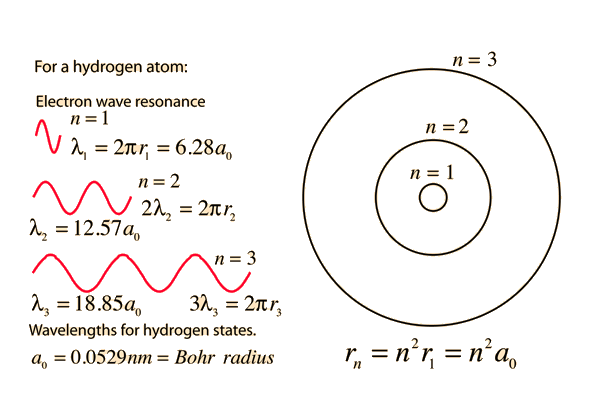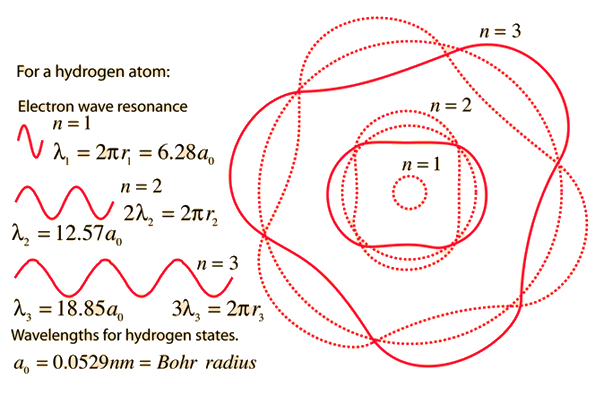Electron Waves and Orbits

Asking why electrons can exist only in some states and not in others is similar to asking how your guitar string knows what pitch to produce when you pluck it. It is a standing wave phenomenon and has to do with resonanceString waves

Index

Bohr model concepts

 HyperPhysics***** Quantum Physics R Nave
Go Back

Electron Wavelengths and Bohr Orbit Radii

The Bohr orbit radius goes up with the square of the principal quantum number n. For orbit n, there are n wavelengths of the electron wave, and these wavelengths are n x the wavelength of orbit n=1.Visualization of waves and orbits Hydrogen orbits
Index

Bohr model concepts

 HyperPhysics***** Quantum Physics R Nave
Go Back

Visualization of Electron Waves

The electron waves for the first three Bohr orbits are visualized here, depicting the waves as meeting a kind of resonance condition so that the continuing waves interfere constructively with each under these conditions. The numbers apply to the hydrogen orbits.Electron waves

Index

Bohr model concepts

 HyperPhysics***** Quantum Physics R Nave
Go Back

The Line Spectrum Problem

In the years before the beginning of the 20th century, the light emitted from luminous gases was found to consist not of a continuous range of wavelengths, but of discrete colors which were different for different gases. These spectral "lines" formed regular series and came to be interpreted as transitions between atomic energy levels. This presented a considerable problem for classical physics, because accelerated charges were known to radiate electromagnetic energy. It was expected that orbits of electrons about positive nuclei would be unstable because they would radiate energy and therefore spiral into the nucleus. No classical model could be found which would yield stable electron orbits.

The Bohr model of the atom started the progress toward a modern theory of the atom with its postulate that angular momentum is quantized, giving only specific allowed energies. Then the development of the quantum theory and the Schrodinger equation refined the picture of the energy levels of atomic electrons.

Bohr model of the atom

Index

Bohr model concepts

 HyperPhysics***** Quantum Physics R Nave
Go Back